## Math in Focus Grade 8 Chapter 3 Lesson 3.3 Answer Key Understanding Linear Equations with Two Variables

Go through the Math in Focus Grade 8 Workbook Answer Key Chapter 3 Lesson 3.3 Understanding Linear Equations with Two Variables to finish your assignments.

## Math in Focus Grade 7 Course 3 A Chapter 3 Lesson 3.3 Answer Key Understanding Linear Equations with Two Variables

### Math in Focus Grade 8 Chapter 3 Lesson 3.3 Guided Practice Answer Key

Complete.

Question 1.
Write a linear equation for the relationship between days, d, and weeks, w. A week has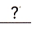days. So a linear equation for d in terms of w is.
Linear equation between days and weeek is
d= 7w,

Explanation:
Assuming there are 7 days in a week, W weeks will have 7 X W days,
So d = 7w.

Question 2.
George rented a car from a company for a week. The table shows the rental charges.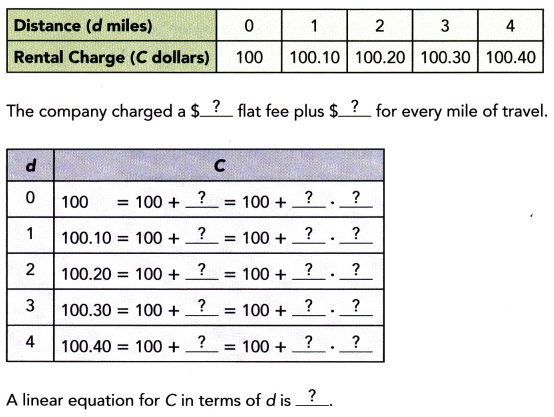A linear equation for C in terms of d is c = 100+(0.1 X d),

Explanation: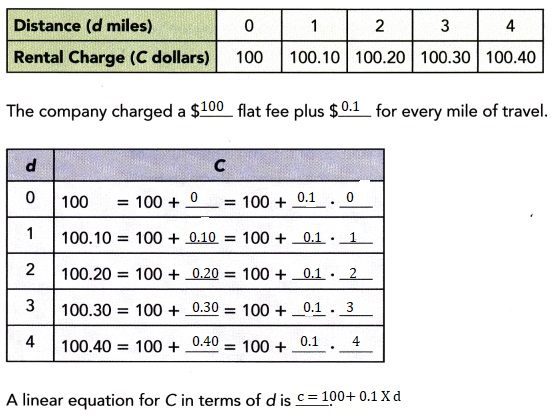Technology Activity

Materials:

• graphing calculator

Use A Graphing Calculator To create Tables Of Values For Linear Equations With Two Variables

Work individually or in pairs.

You can use a graphing calculator to create tables of values for linear equations with two variables. Use the steps to create a table of values for the equation y = $$\frac{x}{\pi}$$. This will give you the diameter y of a circle when you substitute a given circumference x.

Step 1.
Enter the equation using the equation screen.

Step 2.
Set the table function to use values of x starting at 0, with increments of 1.

Step 3.
Display the table. It will be in two columns as shown.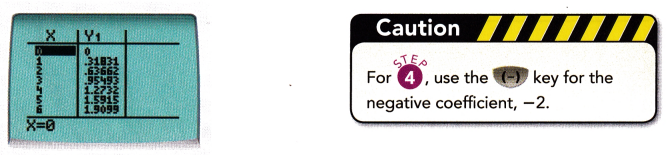Step 4.
Repeat Step 1 to Step 2 for the equation y = -2x + $$\sqrt{2}$$.

Find the value of y when x = -4
9.414,

Explanantion:
When x =-4, y = -2x + $$\sqrt{2}$$,
y = -2(-4) + 1.414
y= 8+ 1.414,
y = 9.414.

Question 3.
y = 7 + 3x
y= -5,

Explanation:
Given y = 7 +3x,
y= 7 +3(-4),
y= 7 – 12,
y= -5.

Question 4.
$$\frac{1}{3} y$$ = 2$$\left(x-\frac{1}{6}\right)$$
y = -25

Explanation: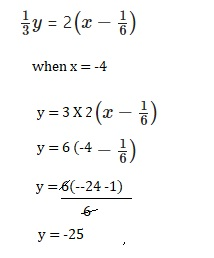Question 5.
-6x – y = 17.75
y = -41.75,

Explanation:
Given -6x – y = 17.75 when x =- 4
-6(-4)-y = 17.75,
-24 -17.75 = y
y = – 41.75.

Create a table of x- and y-values for each equation. Use integer values of x from 1 to 3.

Question 6.
2y = 1.2x + 1
When x =1, y= 1.1, x= 2 , y = 1.7 and when x = 3, y is 2.3,

Explanation: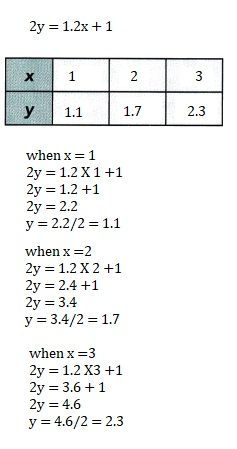Question 7.
4y – 11x = 6
When x =1, y = 17/4 or 4.25,
x= 2, y = 7 and x =3, y = 39/4 or 9.75,

Explanation: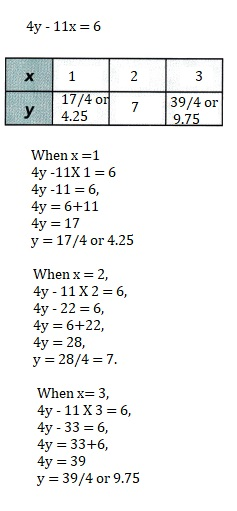Complete the table of x- and y-values for each equation.

Question 8.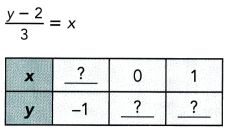When y =-1 x is -1,
when x = 0 y is 2 and
when x =1 y is 5,

Explanation: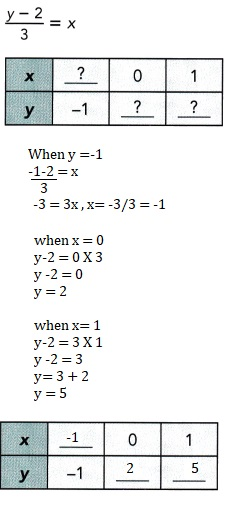Question 9.
3(x + 1) – 2y = 0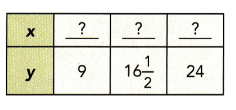y = 9, x is 5, when y =16 1/2, x is 10 and
y = 24, x = 15,

Explanation: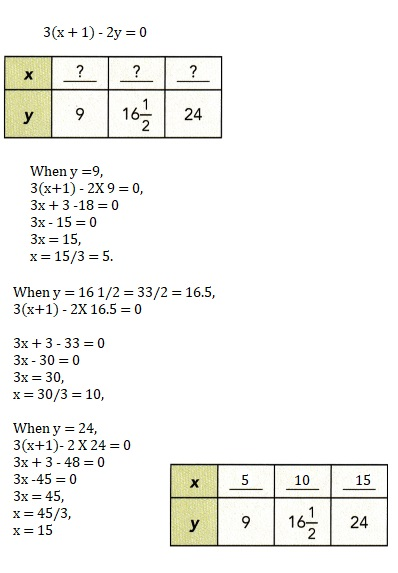### Math in Focus Course 3A Practice 3.3 Answer Key

Write a linear equation for the relationship between the given quantities.

Question 1.
meters, m, and centimeters, c
1 m = 100 c

Explanation:
As 1 meter is equal to 100 centimeters so
1 m = 100 c.

Question 2.
hours, h, and seconds, s
1 h= 3600 s,

Explanation:
As 1 hour is equal to 3600 seconds so
1h = 3600 s.

Question 3.
feet, f, and inches, i
1 f= 12 i,

Explanation:
As 1 foot is equal to 12 inches so
1f = 12 i.

Question 4.
dollars, d, and cents, c
1 d = 100 c

Explanation:
As 1 dollar is equal to 100 cents so
1 d = 100 c.

Find the value of y when x = 2.

Question 5.
2x – 1 = y + 4
y = -1,

Explanation:
Given 2x -1 = y+4, substituting x =2 we get
2 X 2 -1 = y +4,
4 -1 = y +4,
3 = y + 4,
y = 3 – 4 = -1.

Question 6.
y = $$\frac{1}{7}$$(x + 5)
y = 1,

Explanation:
Given y = $$\frac{1}{7}$$(x + 5) and x =2
y = $$\frac{1}{7}$$(2 + 5)= $$\frac{1}{7}$$(7) = 1.

Question 7.
3x – 11 = 2(y – 4)
y = 3/2 or $$\frac{3}{2}$$,

Explanation:
Given 3x – 11= 2(y-4),
3 X 2 – 11 = 2y – 8,
6 -11 +8 = 2y,
14 – 11 = 2y
3=2y,
y = 3/2.

Question 8.
4y = 5(x – 1)
y = 5/4 or $$\frac{5}{4}$$,

Explanation:
Given 4y=5(x-1) and x =2,
4y =5(2-1),
4y = 5(1),
4y = 5,
y = 5/4.

Find the value of x when y = -7.

Question 9.
2(3x – 7) = 9y
x = -49/6 or –$$\frac{49}{6}$$,

Explanation:
Given 2(3x-7) = 9y and y =-7,
6x – 14 = 9 X -7,
6x – 14 = -63,
6x = -63 +14
6x = -49
x= -49/6.

Question 10.
$$\frac{2 x-1}{5}$$ = 2(y + 7)
x = 1/2,

Explanation:
Given $$\frac{2 x-1}{5}$$ = 2(y + 7) when y =-7,
2x -1 = 10(-7+7),
2x – 1 = 0,
2x = 1,
x= 1/2.

Question 11.
2x + y = 0.1(y + 3)
x= 3.3,

Explanation:
Given 2x+y =0.1(y +3) when y=-7,
2x – 7 = 0.1(-7+3),
2x – 7 = 0.1(-4),
2x -7 = -0.4,
2x = -0.4 + 7,
2x = 6.6,
x= 6.6/2 = 3.3.

Question 12.
2y – 5x = 26
y = -9/2 or $$\frac{-9}{2}$$,

Explanation:
Given 2y – 5x = 26, when x = -7,
2y -5(-7)= 26,
2y + 35 = 26,
2y = 26-35,
2y=-9
y = -9/2.

Create a table of x- and y-values for each equation. Use integer values of x from 1 to 3.

Question 13.
y = $$\frac{1}{4}(8-x)$$
x = 1, y = 7/4, x=2, y =3/2 and
when x = 3 y = 5/4,

Explanation: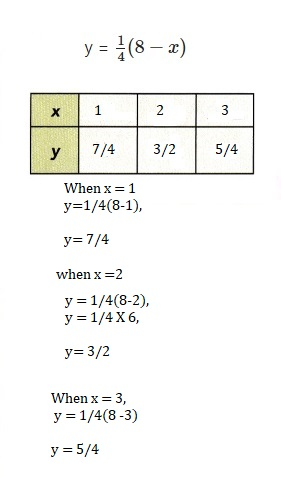Question 14.
x + 7 = $$\frac{1}{2}(y-5)$$
x=1, y = 21,
x=2, y = 23,
x = 3, y =25,

Explanation: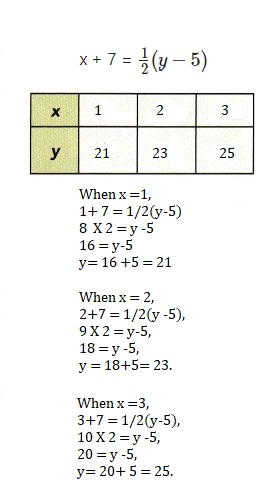Question 15.
-4y = 2x + 5
x = 1, y = -7/4,
x = 2, y =-9/4,
x= 3, y = -11/4,
Explanation: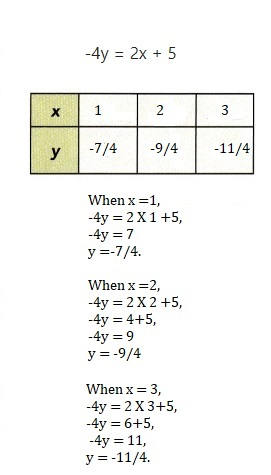Question 16.
$$\frac{1}{2}(x+4)$$ = $$\frac{1}{3}(y+1)$$
x=1, y = 13/2,
x= 2, y =8,
x =3 then y = 19/2,

Explanation: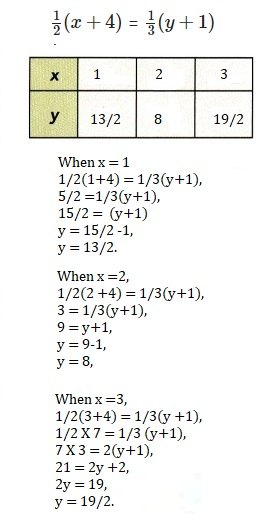Complete the table of x- and y-values for each equation.

Question 17.
y = 5(x + 3)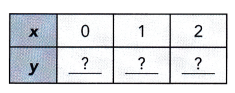x= 0, y =15, x= 1, y = 20, x = 2 , y =25,

Explanation: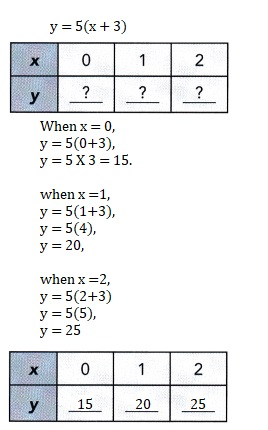Question 18.
$$\frac{x}{4}$$ + y = 1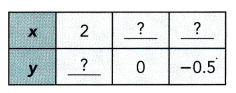x = 2, y = 1/2 or 0.5,
y =0, x = 4 and
when y = -0.5, x = 6,

Explanation: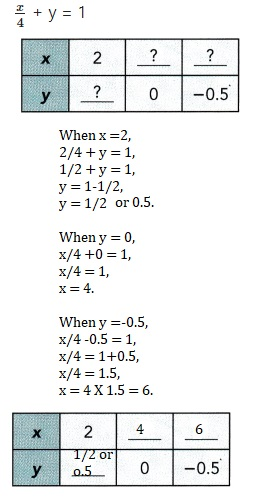Question 19.
3x – 4y = $$\frac{5}{3}$$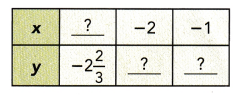y =-2 2/3, x =-3,
x=-2, y =-23/12 and
x = -1 , y = -7/6,

Explanation: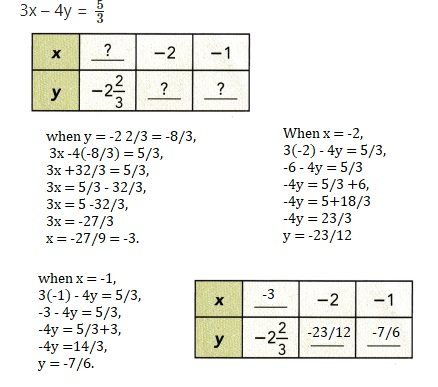Question 20.
5(y + 4) = 8x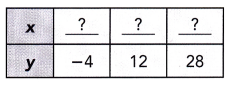y = -4, x = 0, y = 12 , x = 10 and when y = 28 , x is 20,

Explanation: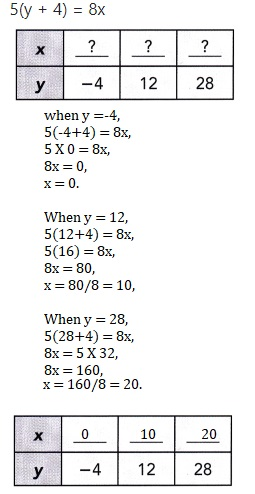Question 21.
A research student recorded the distance traveled by a car for every gallon of gasoline used.
She recorded the results in the following table.
Write a linear equation for the distance traveled,
d miles, in terms of the amount of gasoline used, g gallons.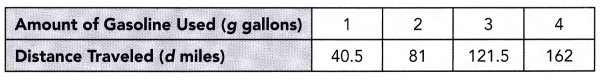d=40.5g,

Explanation:
Given a research student recorded the distance traveled by a car for every gallon of gasoline used.
She recorded the results in the following table. For amount of Gasoline used (g gallons)
for 1 gallons it is 40.5 miles, for 2 gallons it  is 2 X 40.5 = 81 miles,
for 3 gallons it is 3 X 40.5 = 121.5 miles and for 4 gallons it is 4 X 40.5 = 162 miles it is
linear so the equation is d = 40.5 g respectively.

Question 22.
Mr. Taransky sells blood pressure monitors. He earns a monthly salary
that includes a basic amount of $750 and$5 for each monitor sold.
a) Write a linear equation for his monthly salary, M dollars, in terms of the number of monitors sold, n.
M= $750 +$5n,

Explanation:
Given Mr. Taransky sells blood pressure monitors. He earns a monthly salary
that includes a basic amount of $750 and$5 for each monitor sold.
Writing a linear equation for his monthly salary, M dollars, in terms of the number of monitors sold, n.
M = $750 +$5n.

b) Use the equation to complete the table of values below.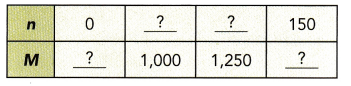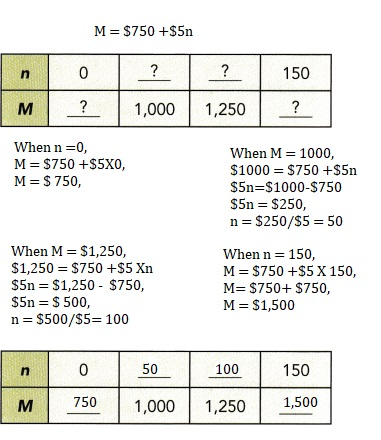Explanation:
Completed the table as shown above.

c) Calculate his salary when he sold 300 monitors in a month.
Mr. Taransky salaray is $2,250 when he sold 300 monitors in a month, Explanation: Given to calculate his salary when he sold 300 monitors in a month, M =$750 +$5 X 300, M =$750 + $1,500 =$2,250.

d) His salary was $1,450 in January. How many monitors did he sell that month? Answer: 140 monitors, Explanation: Given his salary was$1,450 in January, Monitors did he sell that month is
$1,450 =$750+$5n,$5n = $1450-$750,
$5n =$700,
n = 700/5 = 140 monitors.

Question 23.
Bernadette’s cell phone plan costs a basic charge of $20 a month plus 5c per minute of calling time after she uses the first 300 minutes of calling time in a month. a) Write a linear equation for the total cost, C dollars, in terms of the total number of minutes of calling time, t minutes. Answer: Linear equation is c = 20 + 0.05(t -300), Explanation: Given Bernadette’s cell phone plan costs a basic charge of$20 a month plus
5c per minute of calling time after she uses the first 300 minutes of calling time in a month.
The linear equation for the total cost, C dollars, in terms of the total
number of minutes of calling time, t minutes is c = 20 + 0.05(t -300).

b) Create a table of t- and C-values for the linear equation. Use t = 300, 400, and 500.
When t = 300, c = 20, t=400 c = 25 and when t = 500 c is 30,

Explanation: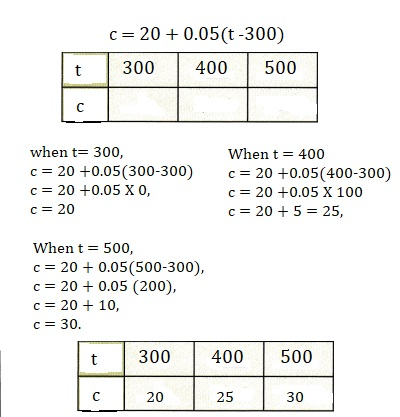c) Find the total number of minutes of calling time Bernardette uses in a month when her bill is $32. Answer: 540 minutes, Explanation: As we have c =20+0.05(t-300) the total number of minutes of calling time Bernardette uses in a month when her bill is$32
$32 = 20 +0.05(t-300),$32 – $20 = 0.05 (t-300),$12 = 0.05(t -300)
t – 300 = 12/0.05 = 240
t = 240 +300 = 540 minutes.

Question 24.
A parking lot charges $1.50 for the first hour or part of an hour. After the first hour, parking costs 70¢ for each half hour, or part of a half hour. Mr. Fischer parked his car in the parking lot for t hours. a) Write a linear equation for the total cost of parking, y dollars, in terms of t. Answer: y=$1.50 + 2(0.7)(t-1)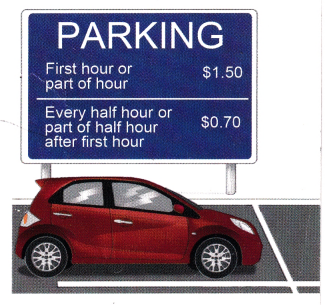Explanation:
Given a parking lot charges $1.50 for the first hour or part of an hour. After the first hour, parking costs 70¢ for each half hour, or part of a half hour. Mr. Fischer parked his car in the parking lot for t hours. linear equation for the total cost of parking, y dollars, in terms of t. y =$1.50 +2(0.7)(t-1).

b) Find the amount Mr. Fischer had to pay if he parked his car for a 40 minutes.
Mr. Fischer has to pay $1.50, Explanation: Given the amount Mr. Fischer had to pay if he parked his car for a 40 minutes as t hours is less than 1 hour, we take t as 1, Mr.Fischer has to pay y=$1.50 +2(0.7)(1-1),
y= $1.50 + 0 y =$1.50.

c) What was the total cost if he parked for 1 hour and 40 minutes?
The total cost if he parked for 1 hour and 40 minutes is $2.90, Explanation: Given the parked time is 1 hour and 40 minutes more than an hour and half hour so we take t as 2, y =$1.50+2(0.7)(2-1) = $1.50 + 2 X 0.7, y=$1.50 +$1.40 =$2.90.

## Math in Focus Grade 4 Chapter 2 Answer Key Estimation and Number Theory

Go through the Math in Focus Grade 4 Workbook Answer Key Chapter 2 Estimation and Number Theory to finish your assignments.

## Math in Focus Grade 4 Chapter 2 Answer Key Estimation and Number Theory

Challenging Practice

Question 1.
The estimated difference between two numbers is 60. Find two numbers that when rounded to the nearest ten, have a difference of 60. Use the numbers in the box.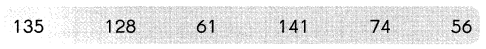After rounding the numbers to the nearest ten, they will be as below.
140        130       60        140       70      60
By seeing the above numbers and to get an estimated difference between any two numbers is 60, the possible combinations will be 130 and 70 (130 – 70 = 60).
So, the two numbers and 130 and 70.

Question 2.
When a 3-digit number is divided by a 1 -digit number, the estimated quotient is 50. Think of two possible numbers that can give this quotient. Then check if your answer is correct.
The three digits numbers are 100, 150.
The one digit numbers are 2, 5.
When a 3-digit number is divided by a 1 -digit number, the estimated quotient is 50.
100/2 = 50
150 /3 = 50

Question 3.
A given number is a multiple of 4. It is between 6 and 1 5. It is a factor of 16. What is the number?
The numbers which are multiples of 4 and between 6 and 15 are 8 and 12.
As it also should be a factor of 16, The required number should be 8.

Question 4.
When a 3-digit number is rounded to the nearest ten and to the nearest hundred, the answer is the same. What is one possible number that fits this rule?
The one possible number that fits the rule is 199.
If 199 rounded to the nearest tens its 200 and rounded to the nearest hundred its 200.

Question 5.
The number of bagels sold each day in two stores follows a pattern. Complete the table below to show this pattern.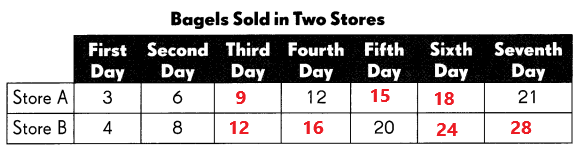Fill in the blanks using the data from the table above.

a. How many bagels did Store B sell on the seventh day? ____
Store B sold 28 bagels on the seventh day.

b. The two stores sold the same number of bagels on different days. Which were the days?
Store A: ____ Store B: __
Store A sold 12 bagels on fourth day.
Store B sold 12 bagels on third day.

Problem Solving

Question 1.
Mr. Chan bought some pencils for a group of students. If he gives them 2 pencils each, he will have 10 pencils left. If he gives them 3 pencils each, he will have none left. How many students are in the group?There are 10 students in the group.
If he give 2 pencils each, that’s 20 pencils, but he has 10 left.
2 x 10 = 20
30 – 20 = 10
If he give 3 pencils each, that’s 30. No pencils left over.
3 x 10 = 30
30 – 30 = 0

Question 2.
On the opening day at a toy store, every third customer gets a ball and every fourth customer gets a stuffed animal. Sixty people come to the store. How many get both a ball and a stuffed animal?
5 people got both a ball and a stuffed animal on the opening day at a toy store.

Question 3.
A square table can seat 4 people. How many square tables are needed to seat 26 people if the tables are put together?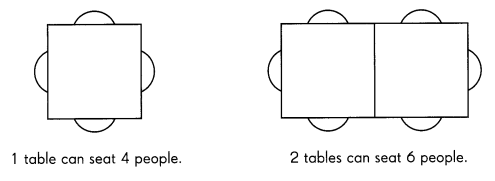A square table can seat 4 people. If the tables are put together, 12 square tables can seat 26 people.

## Math in Focus Grade 5 Chapter 2 Practice 9 Answer Key Real-World Problems: Multiplication and Division

Go through the Math in Focus Grade 5 Workbook Answer Key Chapter 2 Practice 9 Real-World Problems: Multiplication and Division to finish your assignments.

## Math in Focus Grade 5 Chapter 2 Practice 9 Answer Key Real-World Problems: Multiplication and Division

Solve. Use any strategy.

Question 1.
Hannah and Francine have $120. Hannah and Peter have$230. Peter has 6 times as much money as Francine.
How much money does Hannah have?
$98, Explanation: Given information Francine has$X.
Peter has $6X (Peter has 6 times as much money as Francine) Hannah has$Y
X + Y = 120.
6X + Y = 230.

Subtracting above two equations as shown below
(6X + Y) – (X+Y) = 230 – 120
6X + Y – X – Y = 110
Sum: 5x = 110,

X = $22. X + Y = 120, Y = 120 – X = 120 – 22 =$98,
Hannah have $98. Question 2. Larry is 10 years old and his sister is 7 years old. In how many years’ time will their total age be 25 years? Answer: 4yrs, Explanation: Larry = 10 yrs and Sister is 7 yrs, 10 + 7 = 25 – 8, 14 + 11 = 25, After 4 yrs the total age be 25 yrs, Question 3. A box of chalk and 2 staplers cost$10. Three boxes of chalk and 2 staplers cost $1 8. Find the total cost of 1 box of chalk and 1 stapler. Answer: 1 box of chalk =$4 and 1 stapler = $3, Explanation: 1 Chalk Box + 2 Stapler = 10, 3 Chalk Box + 2 Stapler = 18, subtract the above two as shown below to find the cost of 1 box of chalk and 1 stapler (3B+2S) – (B + 2S) = 18 – 10, 3B + 2S – B – 2S = 8, 2B = 8, 1B = 4, B + 2S = 10, 4 + 2S = 10, 2S = 10 – 4 = 6, S= 6/2 = 3. Solve. Use any strategy. Question 4. Sally and Marta had the same number of postcards. After Sally sold 18 of her postcards, Marta had 4 times as many postcards as Sally. How many postcards did each girl have to begin with? Answer: 24 post cards, Explanation: Let the number of postcards with Sally and Marta be X. After selling 18 postcards by Sally, now shelly had X – 18 postcards. Then Marta will have 4 times of Sally. 4(X18) = X, 4X 72 = X, 4X X = 72, 3X = 72, X = 24, Hence each girl had 24 postcards in the beginning. Solve. Use any strategy. Question 5. A basket with 12 apples has a mass of 3,105 grams. The same basket with 7 apples has a mass of 1,980 grams. Each apple has the same mass. What is the mass of the basket? Answer: 405 grams, Explanation: A basket with 12 apples has a mass of 3,105 grams, X Grams per apple, Y Grams = Wt. of basket, 12x + Y = 3105, 7X + Y = 1980, Subtracting the above equation as shown below (12x + Y ) – (7X + Y) = 3105 – 1980, 5X = 1125, X = 225, Replace X with 225, 12X, 12 x 225 + Y = 3105, Y = 3105 – 2700 = 405. ## Math in Focus Grade 5 Chapter 4 Practice 3 Answer Key Multiplying Improper Fractions by Fractions Go through the Math in Focus Grade 5 Workbook Answer Key Chapter 4 Practice 3 Multiplying Improper Fractions by Fractions to finish your assignments. ## Math in Focus Grade 5 Chapter 4 Practice 3 Answer Key Multiplying Improper Fractions by Fractions Complete. Question 1.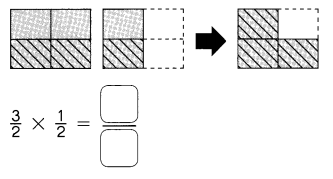Answer: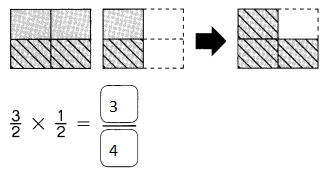Explanation: Multiplication in fractions Numerator is multiplied with numerator and denominator is multiplied with the denominator. Question 2.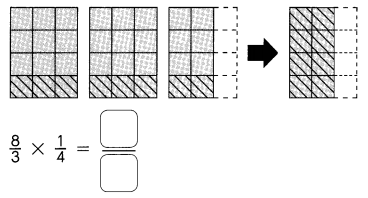Answer: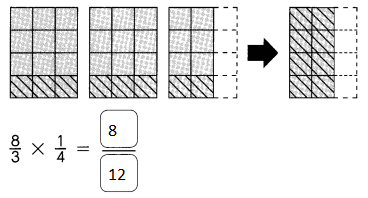Explanation: Multiplication in fractions Numerator is multiplied with numerator and denominator is multiplied with the denominator. Find the product. Question 3.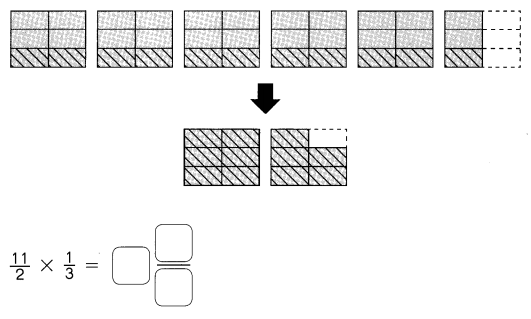Answer: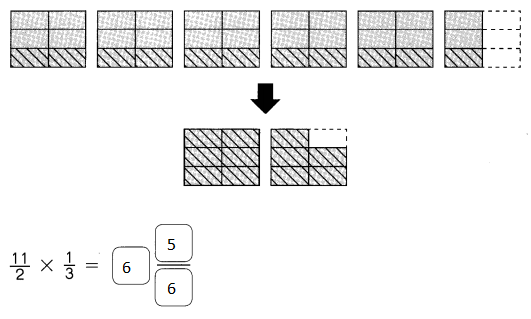Explanation: Multiplication in fractions Numerator is multiplied with numerator and denominator is multiplied with the denominator. Multiply. Express the product in simplest form. Example $$\frac{4}{5}$$ × $$\frac{7}{6}$$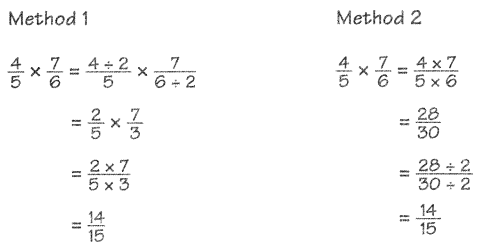Question 4. $$\frac{7}{4}$$ × $$\frac{1}{3}$$ = Answer: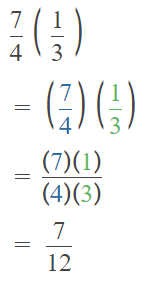Explanation: Multiplication in fractions Numerator is multiplied with numerator and denominator is multiplied with the denominator. Question 5. $$\frac{9}{8}$$ × $$\frac{2}{7}$$ = Answer: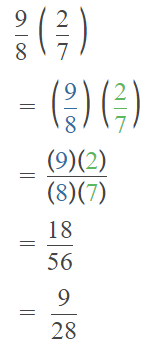Explanation: Multiplication in fractions Numerator is multiplied with numerator and denominator is multiplied with the denominator. Question 6. $$\frac{8}{3}$$ × $$\frac{3}{10}$$ = Answer: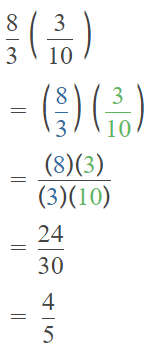Explanation: Multiplication in fractions Numerator is multiplied with numerator and denominator is multiplied with the denominator. Question 7. $$\frac{15}{9}$$ × $$\frac{3}{20}$$ = Answer: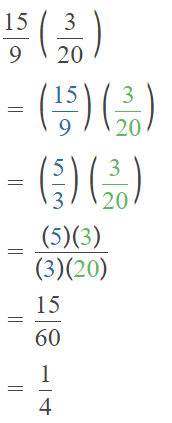Explanation: Multiplication in fractions Numerator is multiplied with numerator and denominator is multiplied with the denominator. Multiply. Express the product as a whole number or a mixed number in simplest form. Example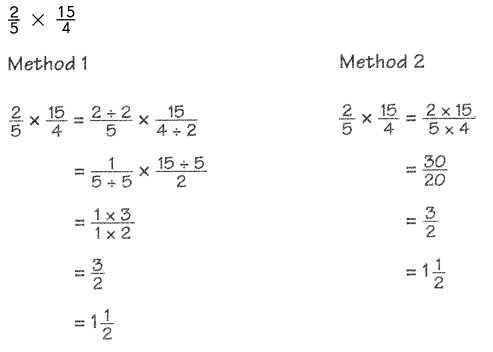Question 8. $$\frac{3}{4}$$ × $$\frac{8}{6}$$ = Answer: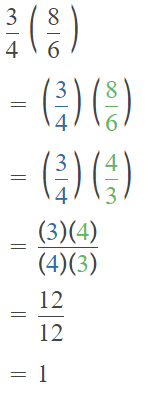Explanation: Multiplication in fractions Numerator is multiplied with numerator and denominator is multiplied with the denominator. Question 9. $$\frac{16}{7}$$ × $$\frac{21}{2}$$ = Answer: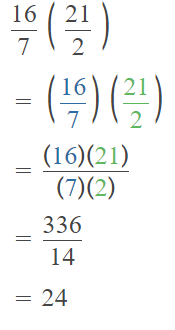Explanation: Multiplication in fractions Numerator is multiplied with numerator and denominator is multiplied with the denominator. Question 10. $$\frac{15}{12}$$ × $$\frac{8}{5}$$ = Answer:Explanation: Multiplication in fractions Numerator is multiplied with numerator and denominator is multiplied with the denominator. Question 11. $$\frac{32}{9}$$ × $$\frac{36}{8}$$ = Answer: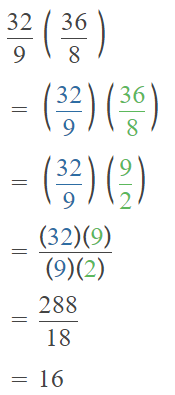Explanation: Multiplication in fractions Numerator is multiplied with numerator and denominator is multiplied with the denominator. Multiply. Express the product as a whole number or a mixed number in simplest form. Question 12. $$\frac{7}{8}$$ × $$\frac{6}{5}$$ = Answer: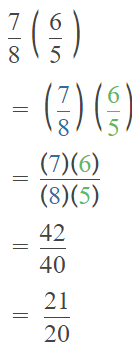$$\frac{7}{8}$$ × $$\frac{6}{5}$$ = 1$$\frac{1}{20}$$ Explanation: Multiplication in fractions Numerator is multiplied with numerator and denominator is multiplied with the denominator. Question 13. $$\frac{11}{12}$$ × $$\frac{28}{3}$$ = Answer: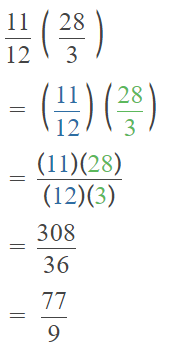Explanation: $$\frac{11}{12}$$ × $$\frac{28}{3}$$ = 8$$\frac{5}{9}$$ Multiplication in fractions Numerator is multiplied with numerator and denominator is multiplied with the denominator. Question 14. $$\frac{21}{5}$$ × $$\frac{15}{6}$$ = Answer: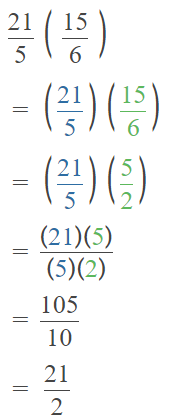Explanation: $$\frac{21}{5}$$ × $$\frac{15}{6}$$ = 10$$\frac{1}{2}$$ Multiplication in fractions Numerator is multiplied with numerator and denominator is multiplied with the denominator. Question 15. $$\frac{25}{4}$$ × $$\frac{18}{10}$$ = Answer: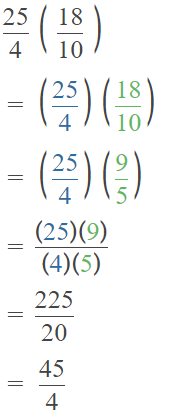Explanation: $$\frac{25}{4}$$ × $$\frac{18}{10}$$ = 11$$\frac{1}{4}$$ Multiplication in fractions Numerator is multiplied with numerator and denominator is multiplied with the denominator. Question 16. $$\frac{30}{9}$$ × $$\frac{7}{2}$$ = Answer: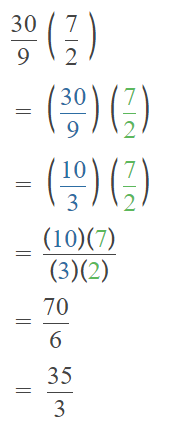Explanation: $$\frac{30}{9}$$ × $$\frac{7}{2}$$ = 11$$\frac{2}{3}$$ Multiplication in fractions Numerator is multiplied with numerator and denominator is multiplied with the denominator. Question 17. $$\frac{14}{8}$$ × $$\frac{5}{3}$$ = Answer: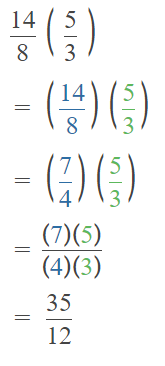Explanation: $$\frac{14}{8}$$ × $$\frac{5}{3}$$ = 2$$\frac{11}{12}$$ Multiplication in fractions Numerator is multiplied with numerator and denominator is multiplied with the denominator. ## Math in Focus Grade 5 Chapter 2 Practice 8 Answer Key Real-World Problems: Multiplication and Division Go through the Math in Focus Grade 5 Workbook Answer Key Chapter 2 Practice 8 Real-World Problems: Multiplication and Division to finish your assignments. ## Math in Focus Grade 5 Chapter 2 Practice 8 Answer Key Real-World Problems: Multiplication and Division Solve. Show your work. Question 1. Rafael has 118 baseball cards arranged in an album. Each page of the album can hold 9 cards. How many pages are full and how many cards are on the last page? Answer: Total 14 pages, Last page has one card, Explanation: Rafael has 118 baseball cards arranged in an album. Each page of the album can hold 9 cards. 118 ÷ 9 = 13 + R 1, Total 14 pages, Last page has one card. Question 2. A ski club had 146 members. Each member paid$30 a month for training fees.
How much did the club collect in fees for the year?
$365, Explanation: A ski club had 146 members, Each member paid$30 a month for training fees.
146 x 30 =  4380,
4380  ÷ 12 = 365,
So, the club collect in fees for the year = $365. Solve. Show Your Work Question 3. A farmer collects 1,250 eggs on a morning. She puts 30 eggs on each tray. How many egg trays does she need to hold all the eggs? Answer: 42 egg trays, Explanation: A farmer collects 1,250 eggs on a morning. She puts 30 eggs on each tray. Total egg trays she need to hold all the eggs 1250 ÷ 30 = 41 + R 20, 42 egg tray needed. Question 4. At a supermarket, pineapple juice sells at$1 per pint (16 ounces).
Greg wants to buy eighteen 40-ounce cans of pineapple juice from the supermarket.
How much does he have to pay altogether?
$45, Explanation: At a supermarket, pineapple juice sells at$1 per pint (16 ounces).
Greg wants to buy eighteen 40-ounce cans of pineapple juice from the supermarket.
1 pint = 16 ounces = $1, 18 x 40 = 720 ounces, 720 ÷ 16 =$45.

Question 5.
A charitable organization spends $4,500 giving out food vouchers to families. a. Each family receives one voucher worth$25. How many families are there?
180 families,

Explanation:
A charitable organization spends $4,500 giving out food vouchers to families. Each family receives one voucher worth$25.
Total number of families $4,500 ÷$25 = 180.

b. Each voucher will be worth $32 next year. How much more money will the charity need next year? Answer:$1,260,

Explanation:
A charitable organization spends $4,500 giving out food vouchers to families. Total number of families = 180, Each voucher will be worth$32 next year
180 x $32 =$5760,
$5,760 –$4,500 = $1,260. Question 6. A group of tourists visits an art museum. The admission is$13 for each adult and $7 for each child. There are 10 adults and 18 children in the group. How much do they pay altogether? Answer:$256,

Explanation:
The admission is $13 for each adult and$7 for each child.
There are 10 adults and 18 children in the group.
$13 x 10 =$130,
$7 x 18 =$126,
$130 +$126 = $256 they pay altogether. Question 7. The length of a rectangular board is 10 centimeters longer than its width. The width of the board is 26 centimeters. The board is cut into 9 equal pieces. a. What is the area of each piece? Answer: 104 square cm, Explanation: The length of a rectangular board is 10 centimeters longer than its width. The width of the board is 26 centimeters. The board is cut into 9 equal pieces. width = w, length = l = w + 10cm, width = 26 cm length = 36 cm total area = 26 x 36 = 936 cm2 936 ÷ 9 = 104 square cm. b. What are the possible dimensions of each piece? (Take the dimensions to be whole numbers.) Answer: 13 X 8 or 8 x 13 possible dimensions of each piece, Explanation: From the above information, length X width = 104 square cm, 13 x 8 = 104 = 8 x 13 possible dimensions of each piece. Question 8. There are 912 yellow chairs and blue choirs altogether in an auditorium. The blue chairs are arranged in 36 rows with 12 chairs in each row. The yellow chairs are arranged in rows of 20. How many rows of yellow chairs are there? Answer: 24 rows, Explanation: 912 yellow and blue chairs in the auditorium. The blue chairs are arranged in 36 rows with 12 chairs in each row. The yellow chairs are arranged in rows of 20. number of yellow rows of yellow chairs are 36 x 12 = 432 blue chairs 912 – 432 = 480 480 ÷ 20 = 24 rows. Solve. Show your work. Question 9. The table shows the wages of workers in Samantha’s company. Sean works from Tuesday through Sunday each week. How much does he earn in 1 week?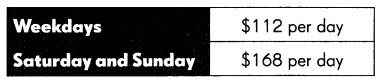Answer:$784,

Explanation:
Sean works from Tuesday through Sunday each week.
The wages of weekdays
4 x $112 =$448,
Wages of Saturday and Sunday
2 x $168 =$336,
Total amount he earn in 1 week
$448 +$336 = $784. Solve. Show Your Work Question 10. The table shows the charges at a parking garage.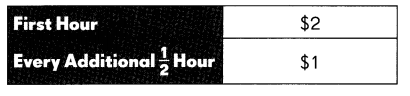a. Sharona parked her car at the garage from 9:30 A.M. to 11:00 A.M. on the same day. How much did she have to pay? Answer:$3,

Explanation:
First hour the parking charges = $2, Every additional half an hour =$1,
Sharona parked her car at the garage from 9:30 A.M. to 11:00 A.M.
9:30 to 11:00 = 1 and half hour is parking time,
$2 +$1 = $3. b. Daryll parked his car there from 9 A.M. to 12:30 P.M. on the same day. How much did he have to pay? Answer:$ 7,

Explanation:
First hour the parking charges = $2, Every additional half an hour =$1,
Daryll parked his car there from 9 A.M. to 12:30 P.M,
9 AM to 12:30 AM = 3 hours and 30 minutes,
=,3 x $2 +$1 = $6 +$1 = \$7.